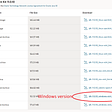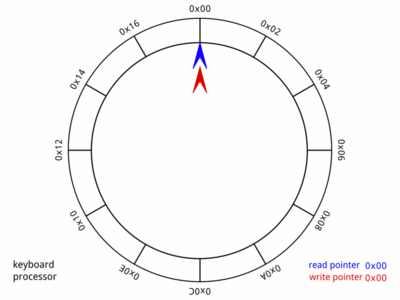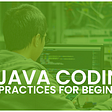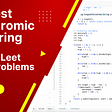# Why do array indexes mostly start at 0?

Have you ever wondered why array indexes start at 0 in most languages? I did. The road to satisfy this curiosity led to a few revelations and fundamentals that we tend to forget a lot of times. Let’s start by defining an array.

Array is typically a contiguous area within the computer memory. In most languages, the variable that we use to refer to this array is inherently a pointer. A pointer that stores the starting address of the contiguous portion of memory.

Let’s take an example, say there’s an integer array `arr` of 5 numbers in a language where every integer occupies 2 bytes of memory. Say, this array's contiguous area starts at byte address 44 and continues until 54 (requiring 10 bytes, i.e. 5 integers of 2 bytes each).

Within this, when referring to `arr[i]` we are essentially looking up the memory location in a shorthand way.

`arr[i] points to a location = Start Address + Data size * Index arr points to a location = 44 + 2*0 = 44 arr points to a location = 44 + 2*1 = 46 arr points to a location = 44 + 2*2 = 48 arr points to a location = 44 + 2*3 = 50 arr points to a location = 44 + 2*4 = 52`

`arr[i] points to a location = Start Address + Data size * (Index - 1) arr points to a location = 44 + 2*(1-1) = 44 arr points to a location = 44 + 2*(2-1) = 46 arr points to a location = 44 + 2*(3-1) = 48 arr points to a location = 44 + 2*(4-1) = 50 arr points to a location = 44 + 2*(5-1) = 52`

We would need to subtract 1 from every index we are accessing if we start indexing at 1. Looking keenly, we are offsetting from starting index to get every element. Using 0 as the index then means using an exact offset from starting point as the index whereas when using 1, we would have to compute the offset itself.

Why use 0 then? Using 0 allows us one less computation for accessing elements. Does this matter that much? Not today, but probably it mattered in the early days of computing to have algorithms in the most efficient way. Maybe, it was an efficiency hack for ancient computers.

Not just this, it also makes mathematical sense to use 0-based indexing. I would not dive much deeper here, but leave it to Dijkstra to take home this point using conventions of representing a sequence of natural numbers.

The simple choice of 0-based indexing simplifies a lot of mathematics on arrays for programmers and allows for some elegant implementations of some concepts like hash tables, consistent hashing, and binary heaps.

Nevertheless, more than a decision of computational efficiency or mathematical accuracy, it is a matter of linguistic choice. With some tweaks in the implementation of the array, you can make any arbitrary indexing work.

Originally published at https://dev.to on April 19, 2022.

--

--

--

## More from Shivam Shekhar

Love podcasts or audiobooks? Learn on the go with our new app.

## Ergo node setup on Windows 10## A Journey of Transformation## Anonymous Authentication Using Power BI## Game Dev Log (2)## Ruby on Rails vs Node.js## Creating my first SlackBot: Dev-Bot## 19 Techniques for Continuous Exploration## Ring buffer data structure at Knorex## Best coding practices every java developer should follow## Leet Code | Longest Palindromic Substring